Courses

# Test: Binomial Numbers (Competition Level)- 1

## 20 Questions MCQ Test Mathematics For JEE | Test: Binomial Numbers (Competition Level)- 1

Description
This mock test of Test: Binomial Numbers (Competition Level)- 1 for JEE helps you for every JEE entrance exam. This contains 20 Multiple Choice Questions for JEE Test: Binomial Numbers (Competition Level)- 1 (mcq) to study with solutions a complete question bank. The solved questions answers in this Test: Binomial Numbers (Competition Level)- 1 quiz give you a good mix of easy questions and tough questions. JEE students definitely take this Test: Binomial Numbers (Competition Level)- 1 exercise for a better result in the exam. You can find other Test: Binomial Numbers (Competition Level)- 1 extra questions, long questions & short questions for JEE on EduRev as well by searching above.
QUESTION: 1

### If 'a' be the sum of the odd terms & 'b' the sum of the even terms in the expansion of (1+x)n, then (1–x2)n equals

Solution:

Sum of odd terms in the expansion of (1+x)n will be 2(n-1) ....(i)
Now
(1−x2)n = nC0nC1 x2 + nC2 x4 + ....(−1)n nCn x2n .....(n)
(1+x2)n = nC0 + nC1 x2 + nC2 x4 +... nCn x2n .....(m)
Subtracting n from m, we get
(1+x2)n − (1−x2)n = 2[nC1 x2 + nC3 x6 + .....]
Now let x=1.
Hence we get
2n − 0 = 2[nC1 + nC3 + .....]
Or nC1 + nC3x2 + nC5 + ... = 2(n-1)
Hence a = b
Now (1−x2)n
=(1+x)n (1−x)n
→[(a+b)(a−b)]
=a2 − b2.

QUESTION: 2

Solution:
QUESTION: 3

### In the binomial (21/3 + 3-1/3)n, if the ratio of the seventh term from the beginning of the expansion to the seventh term from its end is 1/6, then n equals

Solution: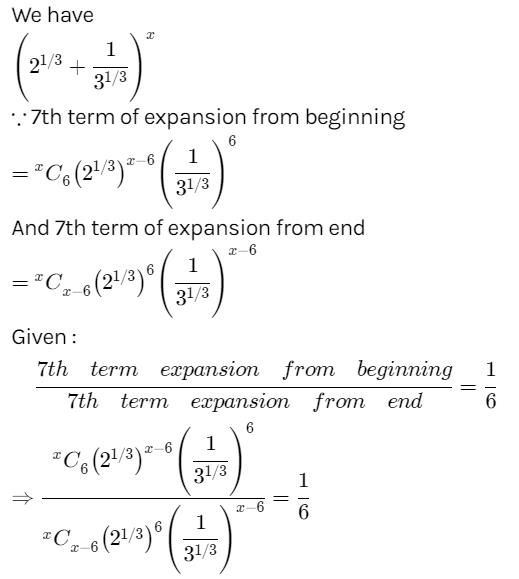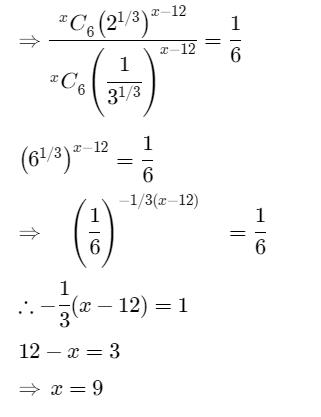QUESTION: 4

If the coefficients of x7 & x8 in the expansion of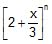are equal, then the value of n is

Solution:

Since Tr + 1 = nCr an – r xr in expansion of (a + x)n,
Therefore,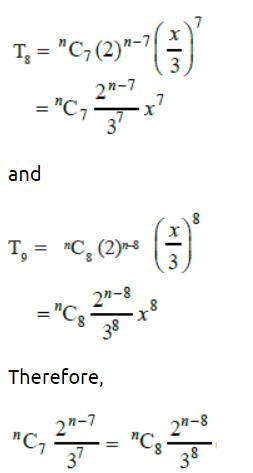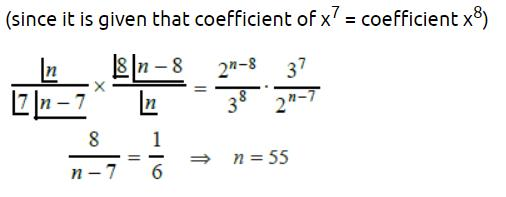QUESTION: 5

The expression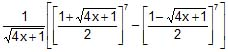is a polynomial in x of degree

Solution:
QUESTION: 6

Number of rational terms in the expansion of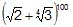is

Solution:
QUESTION: 7

If n ∈ N & n is even, then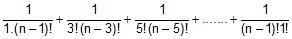equals

Solution:

1/1!(n−1)! + 1/3!(n−3) + 1/5!(n−5) +,
1/n![nC1 + nC3 + nC5 + …………….]
= 1/n![2(n-1)]

QUESTION: 8

The sum of the binomial coefficients of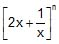is equal to 256. The constant term in the expansion is

Solution:

(2x + 1/x)n
Sum of binomial coefficient = 2n = 256
2n = 28   => n=8
8C4(2x)4(1/x)4
= [(8*7*6*5) * (24* x4-4)]/1*2*3*4
= 1120

QUESTION: 9

The sum of the co-efficients in the expansion of (1 – 2x + 5x2)n is 'a' and the sum of the co-efficients in the expansion of (1 + x)2n is b. Then

Solution:
QUESTION: 10

The number of terms in the expansion of (x + y)n is

Solution:
QUESTION: 11

The greatest terms of the expansion (2x + 5y)13 when x = 10, y = 2 is

Solution:
QUESTION: 12

The binomial expansion of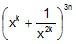, n ∈ N contains a term independent of x

Solution:
QUESTION: 13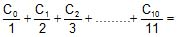Solution:
QUESTION: 14

Let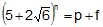where n ∈ N and p ∈ N and 0 < f < 1 then the value, f2 – f + pf – p is

Solution:

p→ integer   f→ fraction
(5+2(6)½)n = p+f  {0<f<1}
f2 – f + pf – p
f(f-1) +p(f-1)
(f+p) (f-1)
(p+f) = (5+2(6)½)n = nC0 5n + nC1 5(n-1)(2(6)½) + nC2 5(n-2)(2(6)½) + nC3 5(n-3)(2(6)½) + ………………….nCn 50 (2(6)½)  --------------------------(1)
0 < (5+2(6)½)n which is approx
(5- 4.8989) = (0.11) < 1
0 <(0.11)n <1
0 <(5-2(6)½)n <1
0 <f’ <1
f’ = (5+2(6)½)n = nC0 5n - nC1 5(n-1)(2(6)½) + nC2 5(n-2)(2(6)½) - nC3 5(n-3)(2(6)½) + ………………….nCn 50 (2(6)½)  --------------------------(2)
p+f+f’ = 2[= (nC0 5n + nC2 5(n-2)(2(6)½) + nC4 5(n-4)(2(6)½) + …………………]
p+f=f’ = 2K    K is integer
f+f’ = 2K-p = integer
f+f’ should be an integer
f2 - f + pf - p
= f(f-1) +p(f-1)
= (f+p) (f-1)   = -(p+f) (1-f)
= -(p+f) (f’)
= - (5+2(6)½)n (5-2(6)½)n
= - {(5+2(6)½)  (5-2(6)½)}n
= - (5)2 - (2(6)2)2
= - [25 - 24]n  => - (1)n
= - 1 (which is negative integer)

QUESTION: 15

If (1 + x + x2)25 = a0 + a1x + a2x2 + .........+a50. x50 then a0 + a2 + a4 + ...........+ a50is

Solution:
QUESTION: 16

The co-efficient of x4 in the expansion of (1 – x + 2x2)12 is

Solution:
QUESTION: 17

If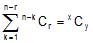then

Solution:
QUESTION: 18

Coefficient of at in the expansion of (a+p)m-1 + (a+p)m-2 (a+q) + (a+p)m-3 (a+q)2 + ....(a+q)m-1 where a >  q and p > q is

Solution:
QUESTION: 19

Number of terms free from radical sign in the expansion of (1 + 31/3 + 71/7)10 is

Solution:
QUESTION: 20

In the expansion of (1 + x)n (1 + y)n (1 + z)n, the sum of the co-efficients of the terms of degree 'r' is

Solution: